# OECD Influenza vaccination rates data analysis with R 6 - multiple regressionGenerated by Bing Image Creator: Orion stars and beautiful forest

This post is following of the above post.

In the previous post, I did simple regression. In this post I will do multiple regressions.

We have 5 explnanatory variables, lgdp, lpc, year, as.factor(year) and as.factor(loc).

I will check two variables combinations among the five variables.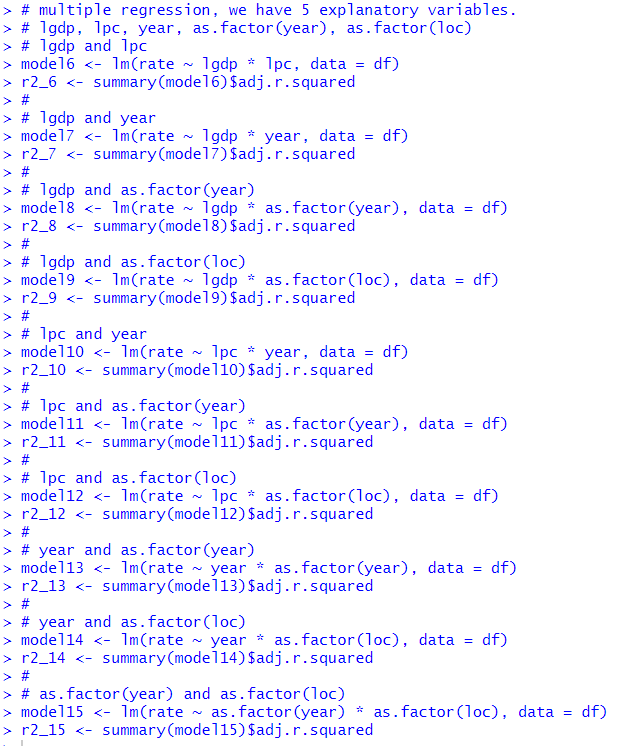I made model6 to model15 and saved those model's adjusted R squared.

Let's comapre them.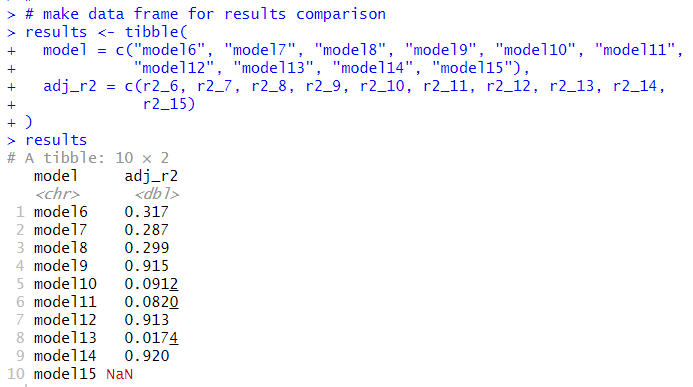modl14 has the largest adjusted R suared. Let's see model14.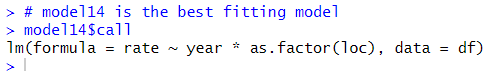model14 explanatory variables are year and as.factor(loc).

Let's visualize the model.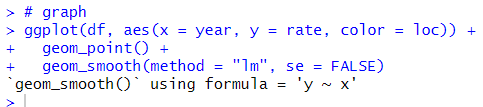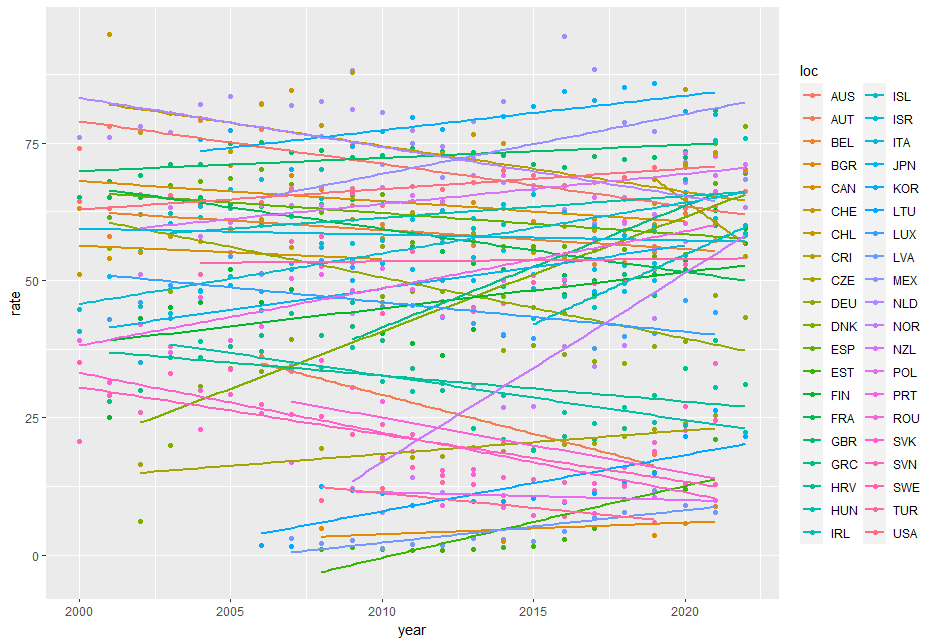That's it. Thank you!

to read from the first post,